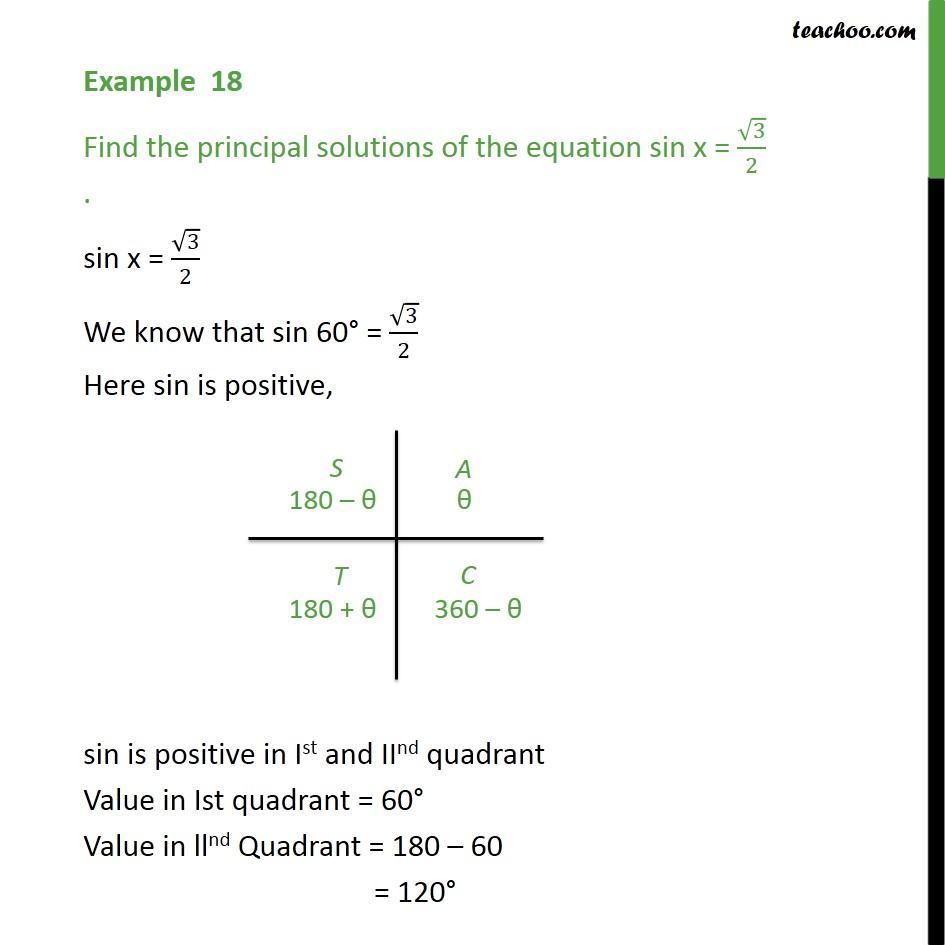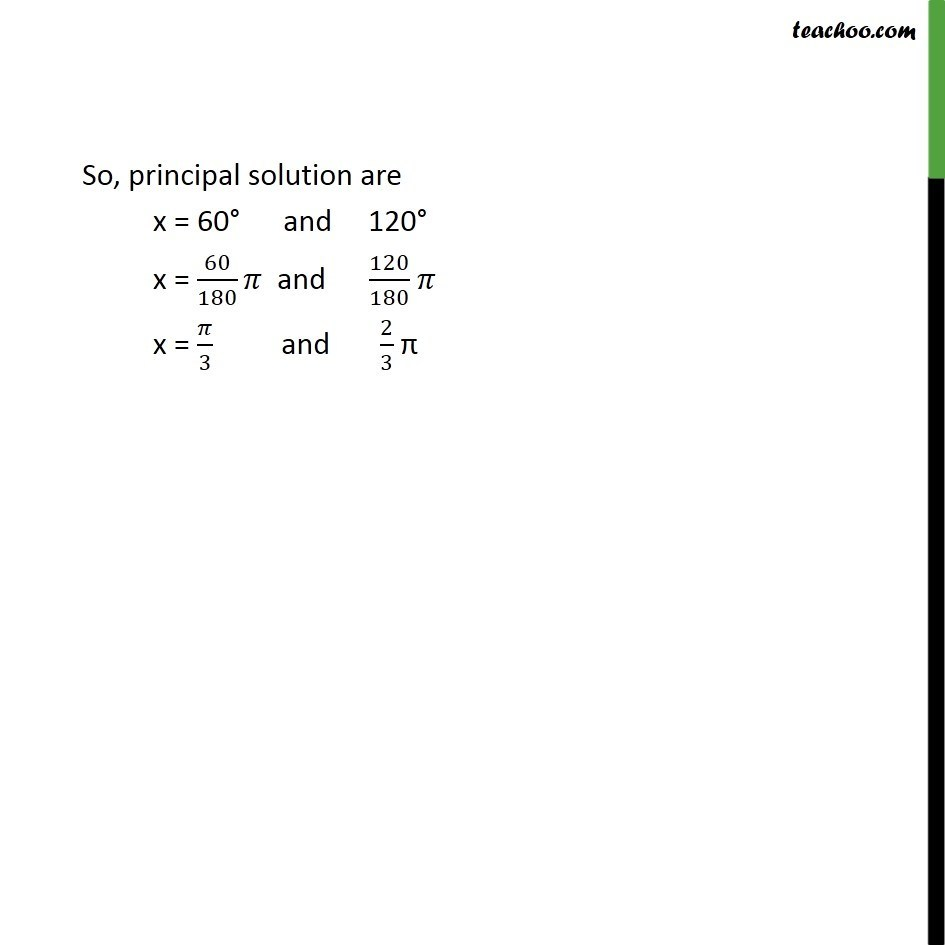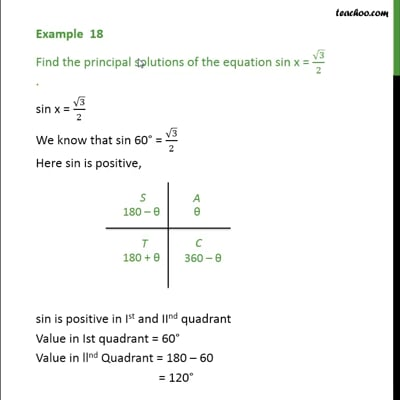Examples

Chapter 3 Class 11 Trigonometric Functions
Serial order wiseThis video is only available for Teachoo black users

Solve all your doubts with Teachoo Black (new monthly pack available now!)

### Transcript

Example 18 Find the principal solutions of the equation sin x = √3/2 . sin x = √3/2 We know that sin 60° = √3/2 Here sin is positive, sin is positive in Ist and IInd quadrant Value in Ist quadrant = 60° Value in llnd Quadrant = 180 – 60 = 120° So, principal solution are x = 60° and 120° x = 60/180 𝜋 and 120/180 𝜋 x = 𝜋/3 and 2/3 π